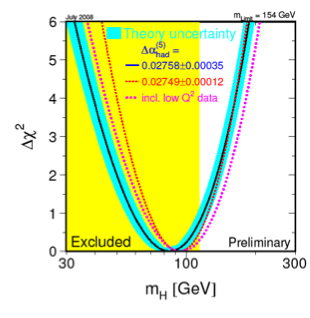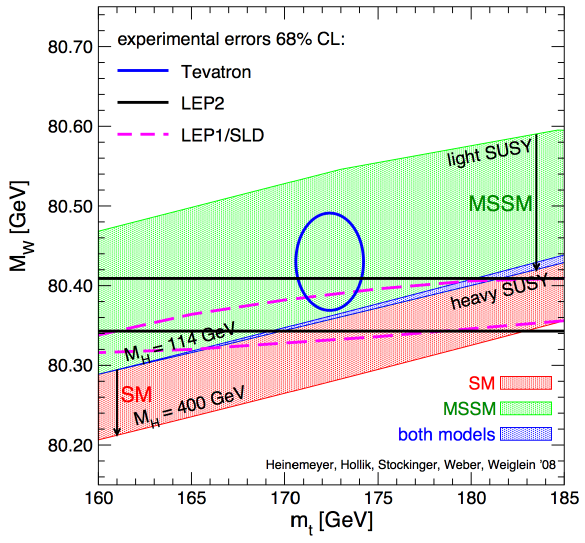## July 31, 2008

### Best Fit

Via Tommaso Dorigo, Pete Renton’s talk at ICHEP08, a report on Global Electroweak Fits and the Higgs Boson Mass. Incorporating the latest Tevatron data,

• $m_t = 172.5\pm 1.2$ GeV.
• $m_W = 80.413\pm 0.048$ GeV.
• $\alpha_s(M_Z^2) = 0.1185 \pm 0.0026$
• $m_H \lt 154$ GeV at the $95\%$ confidence level.
• $m_H = 84^{+34}_{-26}$ GeV (highly sensitive to $m_W$ and $M_t$).The data seem to heavily favour a light Higgs.

The most striking plot, however, from Dorigo’s post, was not in Renton’s talk. It comes from Heinemeyer et al, and the theoretical analysis behind it is explained here.

The red band, corresponding to the Standard Model, comes from varying the only free parameter in the model, $m_H$, from the LEP exclusion bound, $m_H\gt 114$ GeV, up to $m_H=400$ GeV. The green band corresponds to the unconstrained MSSM. The upper edge corresponds to the region where $m_{\tilde{t}_2}/m_{\tilde{t}_1}$ and/or $m_{\tilde{b}_2}/m_{\tilde{b}_1}\gt 2.5$. The lower edge corresponds to the decoupling limit, where all the superpartners are heavy.

The Tevatron seems to mildly favour the MSSM over the SM.While precision electroweak fits are nice, direct search results are nicer. Via Matt Reece, comes news that D0 has excluded (ppt), at 2$\sigma$, $m_H$ in a narrow range centered at 170 GeV. There’s also a summary of the combined CDF/D0 limits on $m_H\gt 155$ GeV.

1 We work in the convention that $m_{\tilde{f}_2}/m_{\tilde{f}_1}\geq 1$

Posted by distler at July 31, 2008 4:34 PM

TrackBack URL for this Entry:   https://golem.ph.utexas.edu/cgi-bin/MT-3.0/dxy-tb.fcgi/1757

## 1 Comment & 0 Trackbacks

### yukawa coupling

The new value of top mass implies thus a yukawa coupling of

y_t= 0.9902 \pm 0.0073

I keep amazed about how the old susy running prediction “y_t has order unity” got a precision two orders of magnitude beyond expectations.

Posted by: Alejandro Rivero on July 31, 2008 5:10 PM | Permalink | Reply to this

Post a New Comment